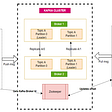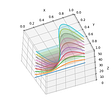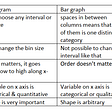# Matrix in Python-Part2 (Operations)

This part will focus only on some special kind of matrices and some very basic operations. These special kind of matrices are used a lot for matrix operations and manipulating them. Most of the code will again go to the same script `matrix.py` so keep it handy.

Initially while starting to write this I considered the special type of matrices to be taken in the end, but these special ones seemed very interesting so I decided to put them on top. So lets start.

But before we enter the realm of square matrices I feel it good to practice some pattern printing codes to sharpen our indexing skills. Also we have testing code in the jupyter notebook and the functions in matrix.py. Both available here.

# Diagonals and Printing Patterns

For printing patterns we will use only square matrices. Why?

Well only square matrices have perfect diagonals. Consider these square matrices in below image.

The Matrix on left is the actual matrix with data and one on right is the matrix to denote row and column indexes.

The numbers crossed with a line denote the diagonal elements or elements on the Main Diagonal. So 1, 5 and 9 lie on the diagonal and have indexes (0,0), (1, 1) and (2, 2) respectively. If you did notice, then there is a relation for elements on main diagonal. Both row and column indexes are same. That is for Mᵢⱼ you have i==j

Before we write code for diagonals lets write a function that will help us a lot in the later sections in creating simple matrices.

This function create_linear_matrix behaves somewhat like the python range function. But here it take dimensions and generate sequence of numbers from 1 till mxn and formats it into a matrix of dimension mxn.

## Printing Diagonals

Now lets write the code to print main diagonal element of the matrix

DIY: Update previous code to print elements in proper diagonal format like this:

`1  5    9`

Coming now to off diagonal. Notice that sum of indexes for off-diagonal elements is 2 in the case below (i.e. size -1 = 3–1 = 2)

So you can replace the condition `i==j` in previous code to this condition `i+j==len(mat) — 1`

## Finding Diagonal Sum

Are you thinking to modify above code and just add a statement to sum the values in the `if i==j `condition? There is a better way to doing that.

Again we will define two functions in matrix.py

Testing code looks like this.

The better way has O(n) time complexity instead of O(n²)

## Printing Triangles

A diagonal splits a matrix into 2 triangles: one above and one below the diagonal. So there can be 4 kinds of triangles as shown below:

Instead of giving you the code for each triangle, I will give you the code to fill in a condition. The condition can be one of these

`i >= j `or` i<=j`or `(i+j)>=(len(mat)-1)` or `(i+j)<=(len(mat)-1)`

Now figure out which condition prints what by substituting conditions in this code:

# Scalar Operations

These are the operations done between a matrix and a scalar(a single number). This involves applying the operation on each element of the matrix with the given scalar value.

This operation is simple and we will just define functions to do scalar addition, subtraction and multiplication straight away. Oh.. actually I will just define addition and leave the rest for you to implement.

And we will define this function a bit differently. Since we can add to the original matrix itself, so our function will take an extra default argument:

inplace = False

This determines whether to modify original matrix and return a None. Or to create a new matrix with the result. With the default option set to create a new matrix.

That’s all about scalar operations. Hey!! but make sure you implement the two functions. We’ll need them later.

# Binary Operations

We will only discuss addition, subtraction and equality of matrices here and leave all rest for next parts.

Equality means each element of one matrix should be equal to the corresponding element at same position in other matrix. And obviously dimensions should match.

Addition and Subtraction are again similar operations just like in case of scalar. Here however for two matrices to be added or subtracted, the dimensions should be same. So our function will return a new fresh matrix as a result with the goodness of both matrices if the dimensions match, otherwise it will return a sad None. See the code below.

# Unary Operations

I think we have gone quite fast and covered a lot of code by now. For this section, we will go a bit slow but obviously there will be more code to see as we go.

# Transpose of a Matrix

Though each section of this series is important, but this one illustrates a special operation on matrices which will allow us to define properties of a matrix like symmetry etc.

Transpose of a matrix is simply interchanging the rows and columns of a matrix. Transpose is an operation which generates another matrix such that its rows become columns and columns become rows.

Transpose of a matrix M is written as Mᵀ. Since rows and columns get interchanged, so an element Mᵢⱼ in M becomes the element Mⱼᵢ in Mᵀ.

Previous code creates a 2 by 3 matrix and then prints its transpose which is a matrix of size 3 x 2. Notice how the row and columns are interchanged. This code however does not change the original matrix mat.

Now lets define a function transpose() that returns a new matrix which contains transpose of original matrix.

There is a special case of square matrices in terms of transpose as you can transpose a square matrix in place. That is you can transform the original square matrix into its transpose, thus saving space. We will see this in the square matrices sections.

## Negative of a matrix

Negative has nothing special to do with it. So just see the code to covert a matrix to another matrix with the sign of all data reversed. Again we will use the inplace option in this as well.

Phew!! That’s it for this part. Find the final matrix.py here

--

--

--

## More from Industrial Python

Lear about Python and the tools used in practical software development

## Short guide: using Nordigen on top of Kontomatik data## Kubernetes learning experience## Big rewards for community contributors## DevOps with GitHub — Part 2: Building and Publishing Packages with GitHub Actions## Message System: Apache Kafka Basics and comparison## Las Vegas Trade Show Models the Story## Step 1 to becoming a software engineer, let’s introduce version control (Part 1)## Creating SOAP Web Service Client in Spring Boot## leangaurav

Developer, Trainer and Fitness & Yoga Enthusiast

## How to plot multiple 2D Series in 3D (Waterfall plot) in Matplotlib## Things you should know about Histogram!## Capital Budgeting in Python